## The Mathematics of PDT Dosimetry for Cancer Treatment

Articles on PDT | PDT front page | Home

Updated Jan 17, 1998 by Steven L. Jacques, Ph.D., Oregon Medical Laser Center
https://omlc.org/pdt/PDTmath/index.html

### This is dry stuff, but someone's got to do the bookkeeping.

This section provides a working description of PDT dosimetry using definitions and expressions presented in Eqs. 1-13. Figure 1 summarizes PDT with a flow diagram.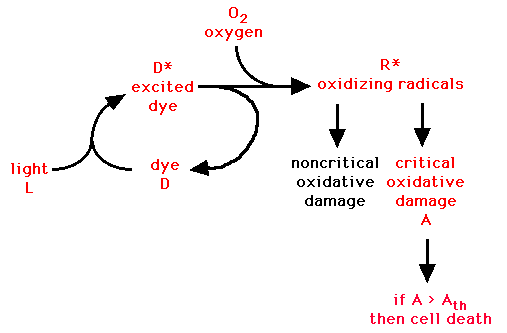Fig. 1: Schematic diagram for PDT. The basic idea: LIGHT + DRUG -> ACTIVATED DRUG + OXYGEN -> OXIDIZING RADICALS -> OXIDATIVE DAMAGE -> CELL DEATH.

## Basics of PDT

As illustrated in Fig. 1, PDT depends on the amount of light delivered (L), the amount of photosensitizing drug (D) in the tissue, and the amount of oxygen (O2) in the tissue. Absorption of light converts D into an activated drug (D*). Reaction of D* with oxygen yields oxidizing radicals (R*, primarily singlet oxygen). A fraction (f) of these radicals attacks critical sites within the cell causing an accumulated oxidative damage (A). When the accumulated damage exceeds a threshold, A > Ath, then cell death occurs.

The light provided by a delivered fluence rate (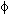) can be expressed in units of photon concentration: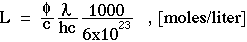(Eq. 1)

whereis the fluence rate of light [W/cm^2] or [J/(cm^2 s)],/(hc) is number of photons per J of energy [ph/J],is the photon wavelength in [cm],
c is the speed of light, 3.0x10^10 [cm/s],
h is Planck's constant, 6.6x10^-34 [J s],
there are 1000 cm^3 per liter,
there are 6x10^23 photons per mole of photons.

The rate constant (k1) for drug activation (D -> D*) is: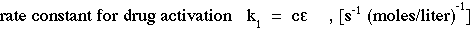(Eq. 2)

where
c is the speed of light [cm/s],is the extinction coefficient of the photosensitizing drug [(cm^-1)/(moles/liter)].

The rate of production of activated drug is: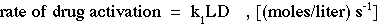(Eq. 3)

The total amount of activated drug (D*) produced per unit volume of tisue is: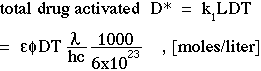(Eq. 4)

where
T is the time of light exposure [s].

The probability that an activated drug (D*) will transfer its excited state energy to oxygen to yield an oxidative radical (R*) is specified by the quantum yield (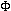) which depends on the oxygen concentration in the tissue. The total amount of R* produced is: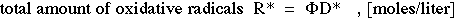(Eq. 5)

A fraction (f) of R* succeeds in oxidatively damaging critical sites in the cell which contributes to cell death. The remaining fraction (1 - f) attack relatively inert or noncritical sites. The accumulation (A) of critical oxidative damage is: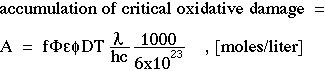(Eq. 6)

If the accumulated damage exceeds a threshold, A > Ath, then cell death occurs.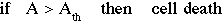(Eq. 7)

## Oxygen

Oxygen is one of the three key ingredients of PDT (drug, light, oxygen). To simplify our mathematical treatment, we have lumped the effects of oxygen concentration into the quantum yieldwhich essentially assumes a constant oxygen availability. However, changes in blood perfusion during PDT can occur which cause O2 to become a variable. Therefore, the dosimetry of oxygen during PDT should be considered when designing a reliable protocol. Spectroscopic monitoring of the oxygen tension of hemoglobin in a tissue is a convenient means of detecting changes in tissue perfusion which is related to oxygen availability. A few laboratories [refs. 2,3] have addressed the dosimetry of oxygen concentration during PDT, and there is a recognized need for more such work.

## Practical dosimetry for the clinic

Currently, the few laboratories concerned with rigorous PDT dosimetry routinely document the light reaching a tissue site (), the amount of photosensitizing drug that accumulates in that tissue site (D), and the light exposure time (T), then calculate the total amount of drug activated during the light exposure period. This factor is quantifiable and therefore a practical dosimetric parameter which has been called the "photodynamic dose" by Patterson, Wilson, Graff [ref. 1]. We have used the symbol D* and the units of [moles/liter] to describe the "photodynamic dose". Patterson et al. have described it as the number of photons absorbed by photosensitizing drug per gram of tissue [ph/g]: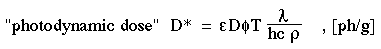(Eq. 8)

where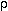is the density of tissue [g/cm^3].

The "photodynamic dose" (D*) does not consider the quantum yield () of oxidative radicals, the effect of oxygen on, or the fraction (f) of radicals that oxidize critical sites. However, "photodynamic dose" is the dosimetric parameter most commonly documented. There is logic in this choice since light (L), drug (D), and exposure time (T) are parameters under experimental or clinical control. Experimental determination of the margins of necrosis induced by a well-defined D* can specify the threshold dose (D*th). The criteria for necrosis is then: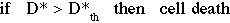(Eq. 9)

For example, a typical D*th for some drugs is about 10^19 [ph/g], which equals a 17 mM concentration of activated drug.

## Treatment zone

For the sake of illustration, consider a treatment using topical irradiation of a tissue surface with a broad beam of light a couple cm in diameter. The light penetration into the tissue can be described by the one-dimensional expression: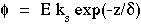(Eq. 10)

where
E = irradiance at tissue surface [W/cm^2],
ks = the backscattering factor which accounts for how reflected light from the tissue augments delivered light [dimensionless],
z = depth into the tissue [cm]= the optical penetration depth [cm], the pathlength which causes the concentration of light to drop to 1/e or 37% of its initial concentration.

Also, assume that the depth of necrosis from such a topical PDT treatment is located at z_necrosis which corresponds to the depth at which the threshold accumulation of oxidative damage, Ath, occurs. Then combining Eq. 9 and 10 and inserting z_necrosis and Ath yields: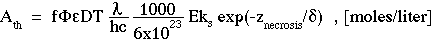(Eq. 11)

Finally, rearrange Eq. 11 to solve for z_necrosis: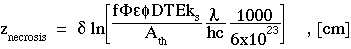(Eq. 12)

Equation 12 shows how the depth of necrosis depends on all the various parameters that affect PDT. Notice that z_necrosis is linearly related to the optical penetration depthbut logarithmically related to all other parameters. To double z_necrosis, one need only doublebut must alter any other factor 7.4-fold.

Again consider the practical dosimetry based on D*, the "photodynamic dose". If the irradiance at the tissue surface yields a D*_surface at the surface, then the depth of necrosis can be expressed: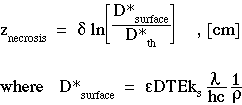(Eq. 13)

For example [adapted from ref. 4], consider the PDT dosimetry using a generic photosensitizer (PS) to illustrate how to estimate the zone of treatment:
 Photons Wavelength630 nm = 630x10^-7 cm Irradiance E 0.2 W/cm^2 = 0.2 J/(cm^2 s) Exposure time T 10 min = 600 s Optical penetration depth0.51 cm Optical backscatter factor ks 4.4 [dimensionless] Conversion constant/hc 3.2x10^18 photons/J Photosensitive drug Administered drug dose - 5 mg/kg body weight = 5 ug/g.tissue Molecular weight of drug MW 600 g/mole Tissue density1 g.tissue/cm^3 Tissue concentration of drug D 3 ug/g.tissue = (3x10^-6 g/g.tissue)(1 mole/600 g)(1 g.tissue/cm^3)(1000 cm^3/liter) = 5x10^-6 moles/liter Extinction coefficient of drug10^4 (cm^-1)/(mole/liter) Quantum efficiency of activating radicals0.1 [dimensionless] "Photodynamic dose" at surface D*_surface 8.4x10^19 ph/g = 143 mmoles/liter Tissue treatment zone Threshold toxic product D*th 10^19 ph/g = 17 mmoles/liter Zone of treatment z_necrosis 1.1 cm

Therefore, the treatment zone, z_necrosis, is a depth of 11 mm for the above generic example of PDT using one-dimensional topical light delivery. To double z_necrosis, one need only doublebut must alter any other factor 7.4-fold.

### References

1 Patterson MS, BC Wilson, R Graff: In vivo tests of the concept of photodynamic threshold dose in normal rat liver photosensitized by aluminum chlorosulphonated phthalocyanine. Photochem Photobiol 51:343-349, 1990.

2 Foster TH, RS Murant, RG Bryant, RS Knox, SL Gibson, R. Hilf: Oxygen consumption and diffusion effects in photodynamic therapy. Radiation Research 126:296-303, 1991.

3 Tromberg BJ, A Orenstein, S Kimel, SJ Barker, J Hyatt, JS Nelson, MW Berns: In vivo tumor oxygen tension measurements for the evaluation of the efficiency of photodynamic therapy. Photochem Photobiol 52:375-385, 1990.

4 Jacques, S.L.: Laser-tissue interactions: photochemical, photothermal, and photomechanical mechanisms. Surgical Clinics of North America, 72:531-558, 1992.

Articles on PDT | PDT front page | Home